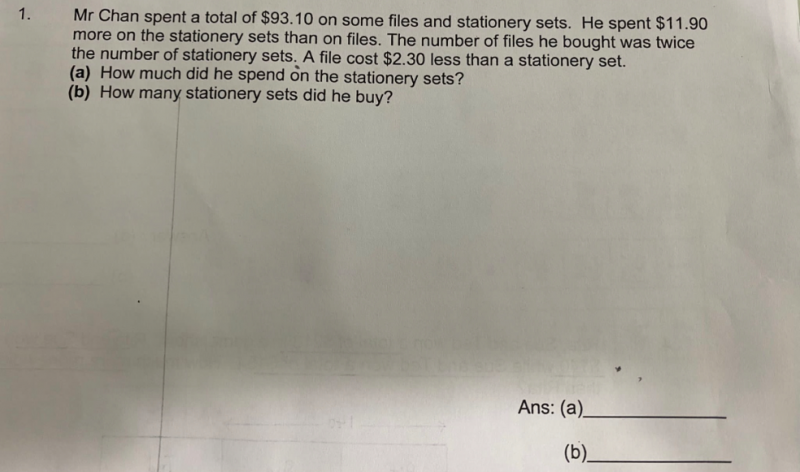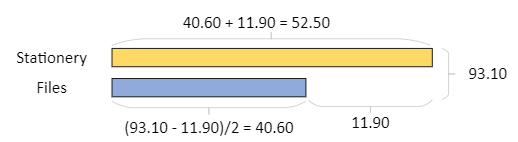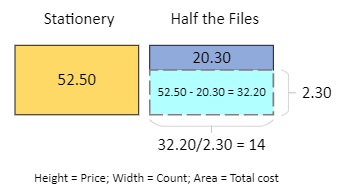# QuestionNo algebra

(a)    Stationery set  [40.60][11.90]  = 52.50                  Total cost = 93.10

Files                   [40.60]                                              (93.10 – 11.90) / 2  = 40.60

Hence cost of stationery sets = 40.60 + 11.90 = \$52.50

(b)   Let u be the number of Stationery Sets bought,  So Files = 2u

Unit Price of File = (40.60) / 2u =  (20.30)/u

Unit Price of  Stationery Set = 2.30  +    (20.30) / u     (SS is \$2.30 more than file)

u  [ 2.30  +    (20.30) / u ] =  52.50             (number of SS x unit price of SS)

2.30u  = 52.50 – 20.30 = 32.20

u = 32.20/2.30  = 14

Hence number of stationery sets bought was 14.

0 Replies 1 Like ✔Accepted Answer```a) Cost of Files = (93.10-11.90)/2 = \$40.60
Cost of Stationery Sets = 40.60 + 11.90 = \$52.50b) We know: 1 Stationery - 1 File = \$2.30.
(Since there are twice as many Files as there are Stationery Sets,
we should half it so that we can compare apple-to-apple.)
Cost of Half the Files = 40.60/2 = \$20.30
Difference in cost = 52.50 - 20.30 = \$32.20
Count of 'Half the Files' = 32.20/2.30 = 14.
So number of Stationery Sets is 14.

Note:
In the 2nd diagram, height of a block represents the price of an item.
Width represents the number / count of items. Thus area of a block
represents the Price x Count = Cost of the items.

No algebra! :D

```
0 Replies 2 Likes